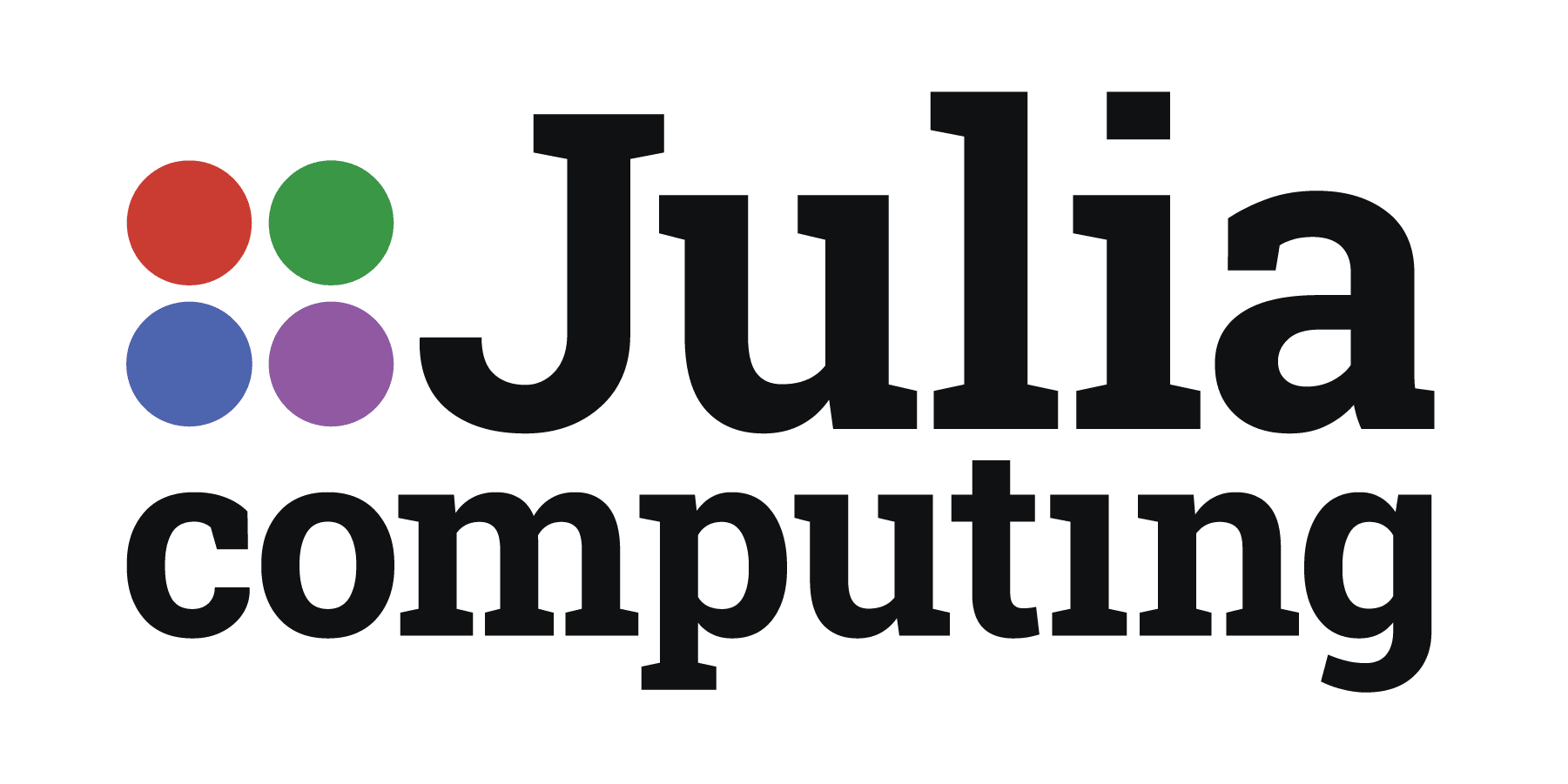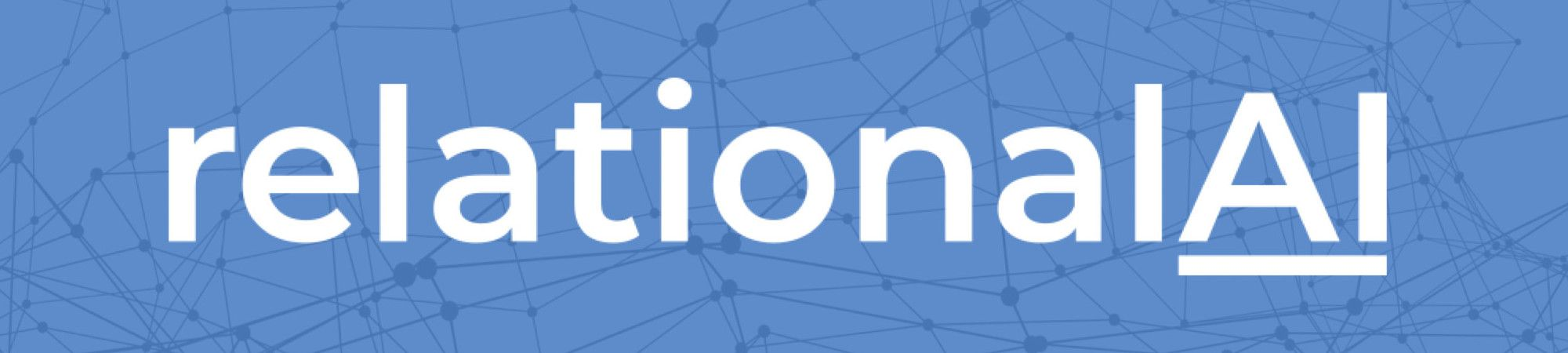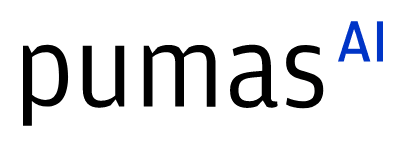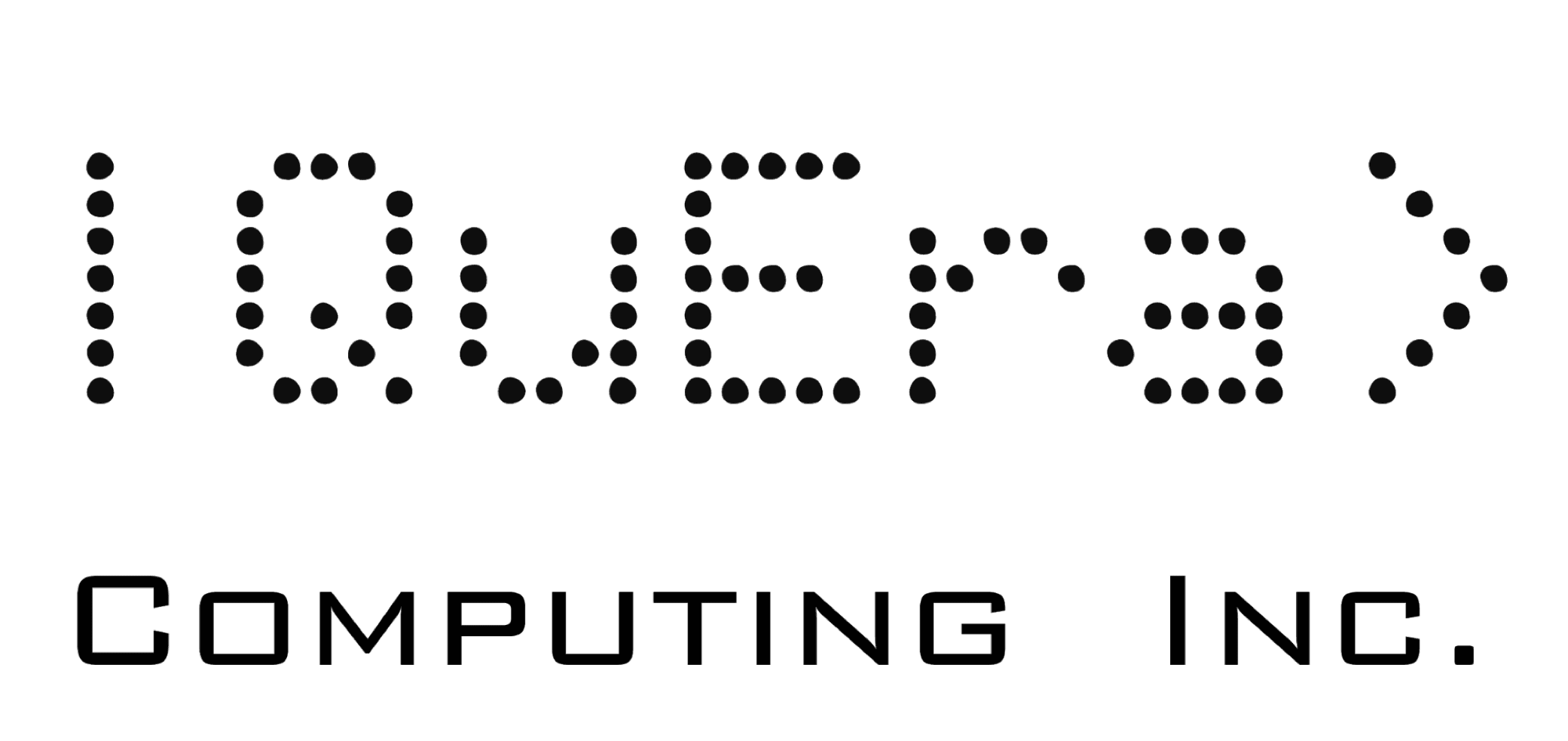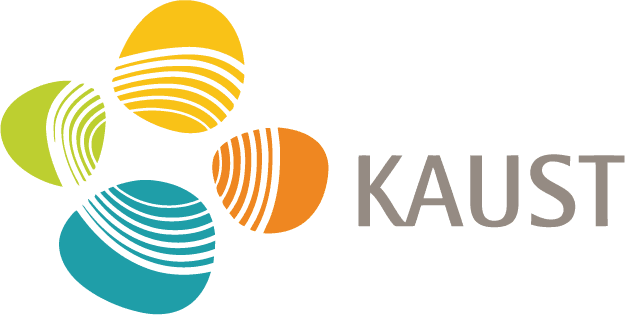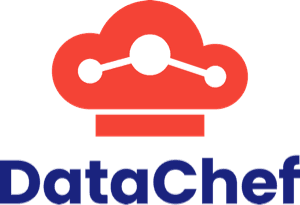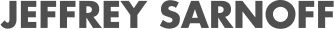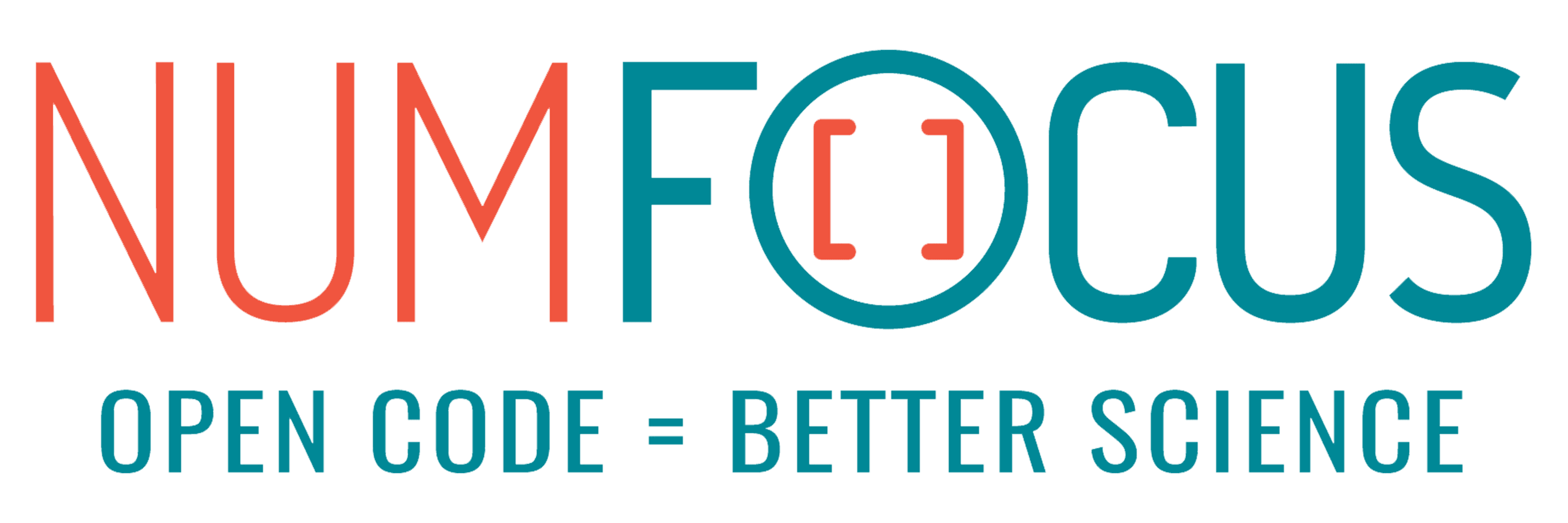# IntervalLinearAlgebra.jl: Linear algebra done rigorously

07/30/2021, 7:40 PM7:50 PM UTC
Purple

#### Abstract:

Solving linear systems is central in most computational domains, from mathematics to engineering applications. This talk will introduce IntervalLinearAlgebra.jl: a package written in Julia to solve linear systems, with interval or real coefficients, rigorously. That is, producing a set guaranteed to contain the true solution of the original problem. This can be applied to solve problems involving uncertainty propagation or perform self-validated computations.

#### Description:

Linear systems arise in practically all domains involving numerical computations. While several efficient floating-point algorithms are available, the final output has no information about how close to the true solution the computed result is. To overcome this, interval arithmetic offers a framework to perform rigorous computations, where real numbers are replaced by intervals guaranteed to contain the true value.

The talk will introduce my Google Summer of Code (GSoC) project: the development of IntervalLinearAlgebra.jl, a package to solve both interval and real linear systems rigorously. During the talk, I will highlight the main features of the package. First, I will give an overview of interval linear systems and demonstrate how to use the package to determine the exact solution, highlighting that even in lower dimensions the solution set can have complex non-convex shapes.

Motivated by this, I will show how to determine a tight enclosure of the solution of an interval linear system, showing the several solution strategies implemented in the package. The presented algorithms will be compared in terms of accuracy and computation time, highlighting the pros and cons of each. During the talk, I will also discuss the lesson learnt during the development process as well as the roadmap beyond GSoC.